# EQ Effects

By now you're familiar with the idea of frequency filters and how emphasizing different frequencies changes and shapes an instrument's tone.

Like filters, equalizers are powerful tools to keep tracks sounding their best and place them properly alongside others in a mix. You can make EQs resonate just like filters or use them as very sensitive tone controls.

Equalizers are used as insert effects, since they "fix" a dry signal to begin with, and mixing a dry and an equalized signal will produce unintentional phasing.

#### The ECF-42 Envelope Controlled Filter

The first EQ effect we'll look at is, in fact, a filter. The ECF-42 is designed to provide the kind of responsive filtering that the SubTractor's filter module allows, but is available to any instrument that is connected to it.

The simplest way to use it is to connect it to an audio source and use it as an insert filter effect. When cabled with audio inputs and outputs only, the gate, velocity, and envelope amount controls will have no effect, and the ECF-42 will act as a regular filter.

#### To use the ECF-42 as a straight resonant filter

1.

Select the instrument you want to use as a signal source and choose Create > ECF-42 Envelope Controlled Filter (Figure 8.73).

##### Figure 8.73. We'll use the ECF-42 Envelope Controlled Filter as a straight resonant filter.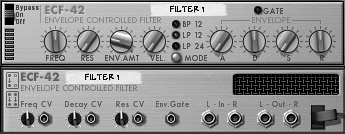If you're hooking up a mono source like a SubTractor synth, only the left inputs and outputs will be used (Figure 8.74).

##### Figure 8.74. With the ECF-42 used as a mono effect, only the left inputs and outputs are used.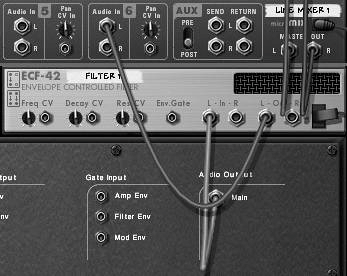2.

Use the ECF-42 Res knob to add resonance.

3.

Use the Freq knob to set the filter frequency.

Now for the "envelope-controlled" part. To use the filter's envelope generator, you'll need to supply a gate CV signal to the ECF-42's Gate CV input.

In this example we'll use one Matrix to play a SubTractor and another to play the ECF-42. The result is one pattern for the instrument, and a second pattern for the filter.

#### To set up two pattern sequencers

1.

Create a SubTractor synth.

2.

Set the Filter 1 Freq fader to maximum and set both the F.Env and F.Dec velocity knobs to the middle (Figure 8.75).

##### Figure 8.75. Set up the SubTractor so it produces no filter activity.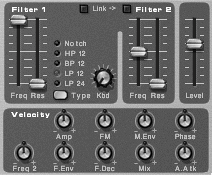This is so we don't have any filter activity from the SubTractor.

3.

Select the SubTractor and create a Matrix Pattern Sequencer.

This will automatically connect the Note outputs and Gate CV of the Matrix to Gate and CV inputs of a SubTractor (Figure 8.76).

##### Figure 8.76. Connect a Matrix to the SubTractor.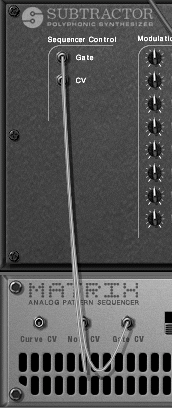4.

With the SubTractor selected, create an ECF-42.

Reason will insert the ECF-42 between the SubTractor and the Mixer (Figure 8.77).

##### Figure 8.77. Set up an ECF-42 as an insert effect.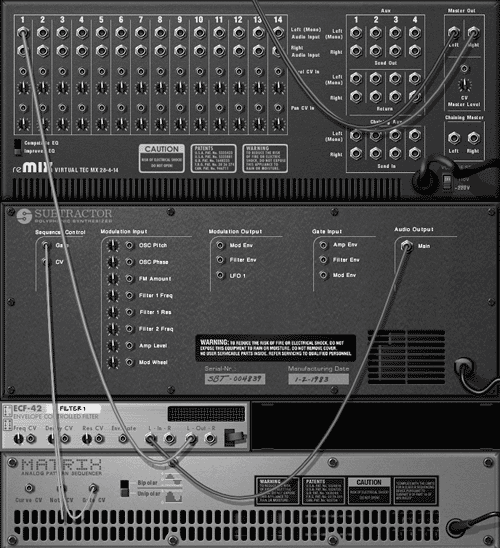5.

Select the ECF-42 and create a second Matrix.

Reason will route the Gate CV output of the new Matrix to the Env Gate CV input of the ECF-42 (Figure 8.78). Once a gate signal is patched to the ECF-42, you'll have extra controls, which function just like the velocity sensitivity controls of the SubTractor.

##### Figure 8.78. Connect a second Matrix to the ECF-42.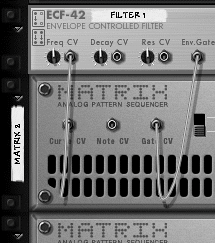6.

In the Matrix controlling the SubTractor write a short bass loop (Figure 8.79).

##### Figure 8.79. Create a Matrix bass loop for the SubTractor.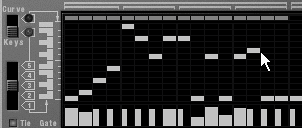#### To control the ECF-42 with Matrix velocity

1.

Draw velocity variations in the Gate lane of the second Matrix (Figure 8.80) and click Run.

##### Figure 8.80. A gate track in the Matrix will drive the ECF-42 filter.Higher gate values will make the sound brighter.

2.

Turn the ECF-42 Freq knob all the way to the left (Figure 8.81).

##### Figure 8.81. Turning the ECF-42 Freq knob to the left leaves room for the Matrix to open the filter with high velocities.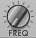Starting the filter at a closed position gives the Matrix room to open it up.

3.

To begin filter motion, turn the ECF-42 Vel knob to the right (Figure 8.82).

##### Figure 8.82. To start motion, turn the ECF-42 Vel knob to the right.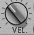The ECF-42 will start varying the brightness of the SubTractor. The Vel knob determines how far the filter will open in response to velocity increases.

4.

Boost the ECF-42 Res knob for the desired amount of resonance.

5.

Continue to balance the Freq and Vel knobs until you have the desired sound.

Low Vel settings mean that the filter is moving less, so higher Freq settings may be necessary.

High Vel settings move the filter more a lower Freq setting can create a mute effect, which makes the high-velocity notes more striking.

Now we'll use these settings to move the envelope.

#### To control the ECF-42 envelope

1.

With your Matrix devices running, boost the ECF-42 Env.Amt knob to about halfway (Figure 8.83).

##### Figure 8.83. Turn the Env.Amt knob to about halfway.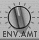2.

Now try decreasing the envelope D knob to about 44 (Figure 8.84).

##### Figure 8.84. Short envelope decays can sound "bubbly."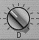This will shorten the decay and make the SubTractor line sound as if it's bubbling.

3.

Try boosting the A knob to fade in high frequencies during note attacks (Figure 8.85).

##### Figure 8.85. Boost the A (attack) knob for a rapid fade-in.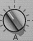4.

Turn the A knob hard left (off) and the D knob left to about 32, then start increasing the S knob.

Unless A and D are set very low, the S knob has little effect on rapid notes.Tip

• For a quick refresher on ADSR and filters, see the "Filter Envelope" section of Chapter 5, "Building a Song."

Now let's set a pattern in a Sequencer track and control the ECF-42 that way.

#### To control the ECF-42 from a sequencer track

1.

Continuing with the setup from the previous task, delete the second Matrix.

This will mute the sound temporarily.

2.

In your Sequencer, choose Create > Sequencer Track.

3.

Assign your new Sequencer track to the ECF-42 (Figure 8.86).

##### Figure 8.86. Assign a new Sequencer track to control the ECF-42.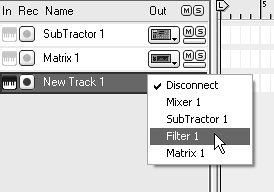4.

Switch to Edit mode and input a series of notes (Figure 8.87).

##### Figure 8.87. This series of notes is designed to drive the ECF-42 gate.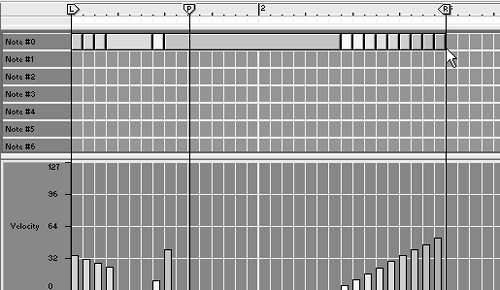This is a gate track, so there's no need to write melodies.Tip

• You can also control any CV input on the ECF-42 from the Sequencer track's Controller lane (Figure 8.88), or from another device by patching CV outputs from it to any of the ECF-42's CV inputs.

##### Figure 8.88. Control the ECF-42 frequency using the Controller lane.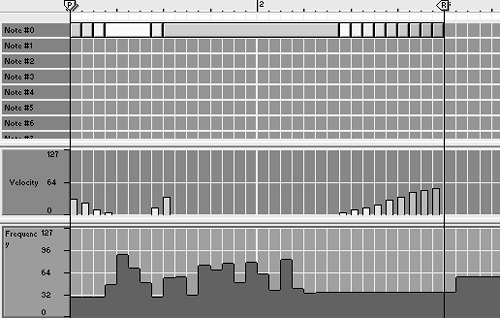Reason 3 For Windows and Mac
ISBN: 321269179
EAN: N/A
Year: 2003
Pages: 180